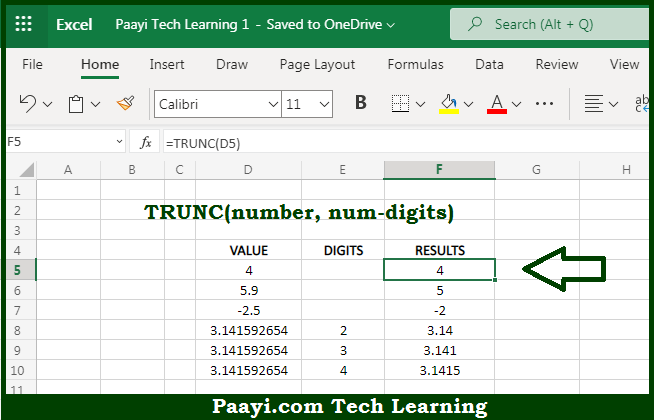# Learn How to Use Microsoft Excel TRUNC Function

Written by | 0 Comments | 501 Views

In this article, you will learn how to use the Microsoft Excel TRUNC function and its prime function in Microsoft Excel. You will also get to know the Microsoft Excel TRUNC function return value and syntax with the help of some examples.

Microsoft Excel TRUNC Function

The main function of the Microsoft Excel TRUNC function is to get the truncate of numbers to a given precision. That implies, with the help of the TRUNC function you can able to get a truncated number, that is based on the optional number of digits. It should be noted that TRUNC(5.9) will return 5, and TRUNC(-4.5) will return -4. Also, the TRUNC function is not used for rounding, it simply truncates as specified. So, with the help of the TRUNC function, you can able to get the truncate of the numbers to a given precision.

Return Value of TRUNC Function

The return value will be the truncated number to the given precision.

Syntax of TRUNC Function

=TRUNC(number, [num-digits])

Where the arguments:

• number: This is the number that you want to truncate.
• num-digits: This is the precision of the truncation, defaults to 0 (optional).

How to Use Microsoft Excel TRUNC Function?So we know that Microsoft Excel TRUNC function you can able to get the truncate of numbers to a given precision. That implies, with the help of the TRUNC function you can able to get the truncated number, which is based on the optional number of digits. It should be noted that TRUNC(5.9) will return 5, and TRUNC(-4.5) will return -4. Also, the TRUNC function is not used for rounding, it simply truncates as specified. So, with the help of the TRUNC function, you can able to get truncate of numbers to a given precision.